# BASE Function

Converts a number into the supplied base

## What is the BASE Function?

The BASE function is available under Excel Mathematical and Trigonometric functions. The function returns a text representation of the calculated value and will convert a number into the supplied base (radix). BASE was introduced in Excel 2013 and is unavailable in earlier versions.

### Formula

The BASE function uses the following arguments:

1. Number (required argument) – This is the number that we wish to convert. The number should be an integer and greater than or equal to 0 and less than 2^53.
2. Radix (required argument) – The base radix is what we want to convert the number into. It must be an integer greater than or equal to 2 and less than or equal to 36. Radix is the number of unique digits, including zero, used to represent numbers in a positional numeral system. For example, for the decimal system, the radix is 10, as it uses ten digits from 0 to 9.
3. Min_length (optional argument) – This is the minimum length of the string returned. If specified, it must be an integer greater than or equal to 0.

### How to use the BASE Function in Excel?

To understand the uses of the BASE function, let’s consider a few examples:

#### Example 1

Suppose we want to convert the number 10 to base 2: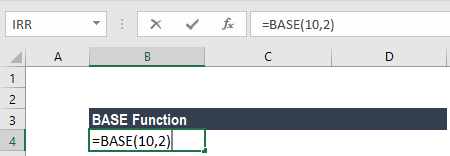The BASE function will convert the decimal number 10 to base 2 (binary system) and give us the following result: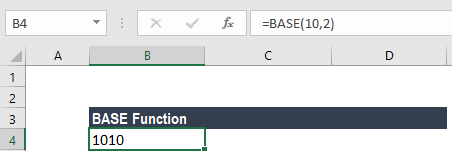On a similar basis, we can give different radix to get the desired output. A few examples are shown below: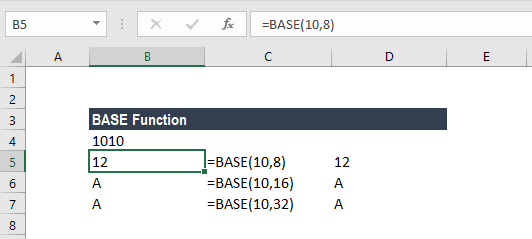#### Example 2

Now, let’s see how this function behaves when we specify a minimum_length argument. Suppose we specify the number 12, with radix 2 and minimum length 10: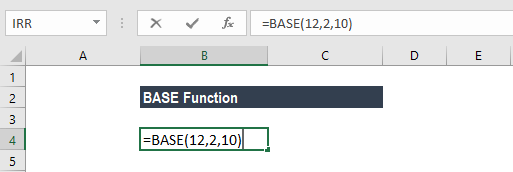In the example, the function will convert the decimal number 12 to base 2 (binary), with a minimum length of 10. The result would be 0000001100, which is 1100 with 6 leading zeros to make the string 10 characters long.

### Few notes about the BASE Function

1. #VALUE! error – Occurs when the number given is a non-numeric value.
2. #NUM! error – When any of the argument that is number, radix, or min_length is outside the minimum or maximum constraints. So, the error will occur:
• When the given number argument is < 0 or is ≥ 2^53.
• When the given radix argument is < 2 or > 36.
• If the [min_length] argument is supplied and is < 0 or ≥ 256.
3. #NAME! error – Occurs when the formula contains an unrecognized value in any of the arguments.
4. If we enter a non-integer number, the argument is truncated to an integer.
5. The maximum value of the min_length argument is 255.
6. If we enter the min_length argument, leading zeros are added to the result if the result would otherwise be shorter than the minimum length specified. For example, BASE(32,2) returns 100000, but BASE(32,2,8) returns 00100000.
7. The function can also be used by specifying a cell reference. If the cell referred to is empty, it will take the number as zero.

For example, if we give the formula =BASE(A3,2,10), wherein A3 is the cell referred to and is empty. In such a scenario, the BASE function will return the following result as A3 is empty.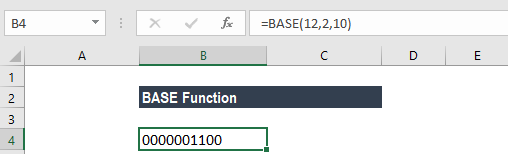Thanks for reading CFI’s guide to important Excel functions! By taking the time to learn and master these functions, you’ll significantly speed up your financial analysis. To learn more, check out these additional CFI resources:

• Excel Functions for Finance